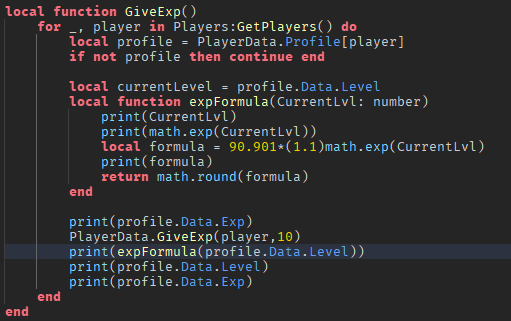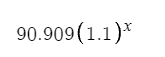# Why is my math not mathing

So I’m having a problem with, I’m assuming with math.exp. It keeps making my math equal to 100 (which is correct IF the level is set to 1, which is not) I checked my arguments to see if it was set to one or something but that wasn’t the case. Any Help will be appreciated!14 Likes

You didn’t math enough. Needs more math. You need the math for the math of the math to complete the math with the math.

The Lua function `math.exp(x)` returns the value of `e` raised to the power of `x`. In other words, it returns the exponential value of `x`. For example, `math.exp(1)` returns the value of e (`2.71828`) raised to the power of 1 which is approximately `2.71828` 1 2 3.

Edit-
I think what I said here was completely relevant to the original topic. Remember people, think smarter and not harder, i.e. don’t over-complicate (GOA7) haha. I’ll explain why.

If you plug `1` into `math.exp` it’ll return this mess → `2.71828`. If you input `2` into the function instead, it’ll return with this even bigger mess → `7.3890560989306495`. Times that by `1.1` and you get this even bigger mess than the last `8.128961708823714`. Now multiply that number by `90.9` and you get `739.68`. Perhaps I mathed enough here and knew what I was talking about? I can’t believe I needed to explain this.5 Likes

It seems like you didnt math enough here.

Thats Literally not what he is asking, and the AI response is not helpful here, so please provide relevant information.

You Arent Adding `math.exp()` to the Variable, because of that its instead firing it separately from said variable, thats why you are only getting `100`, so probably tell the variable that its: `x + e^y`, or in code:

``````local formula = 90.901*(1.1) + math.exp(CurrentLvl)
``````

(assuming you are adding, otherwise multiply it)

2 Likes

I’m trying to make this equation right here1 Like

then in this case you would use the operator `^` for this so `x^(e^y)` would be what you want, otherwise you use `math.pow()` which would return the exact same result.

You could try a simpler route where you simply set the new max exp based upon the user’s level, like

``````local maxExp = currentLevel * 12
-- 1 * 12 = 12
-- 2 * 12 = 24
-- 3 * 12 = 36
-- etc
``````

It’s a lot simpler and more readable. Takes out the fuss as well. Ez solution

Im only trying to do 10% of max in my case

1 Like

The operator works like a charm. Thank you!

1 Like

Do you mean in multiples of `10` above `100`? If so, that should be fairly simple.

``````local maxExp = 100 + (currentLevel * 10)
``````

.

No problem, haha. Sometimes the simplest solution is the best, unless you’re trying to be b i g b r a i n and show off to the ladies.1 Like

Wait that works too lol. Thanks for the insight!

This topic was automatically closed 14 days after the last reply. New replies are no longer allowed.